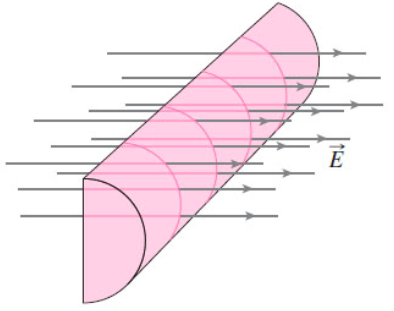# Problem: In (Figure 1 take the half-cylinder's radius and length to be 3.4 cm and 15 cm, respectively. If the electric field has a magnitude of 5.9 kN/C. Find the flux through the half-cylinder. Hint: You don't need to do an integral! Why not?Express your answer with the appropriate units.

###### FREE Expert Solution

The flux is given by:

$\overline{){\mathbf{\varphi }}{\mathbf{=}}{\mathbf{\int }}{\mathbf{E}}{\mathbf{·}}{\mathbf{d}}{\mathbf{A}}}$, where E is the electric field and dA is the area element.

In this case, the dot product is unnecessary since the area vector is pointing in the direction of the electric field vector E.

94% (38 ratings)###### Problem Details

In (Figure 1 take the half-cylinder's radius and length to be 3.4 cm and 15 cm, respectively.If the electric field has a magnitude of 5.9 kN/C. Find the flux through the half-cylinder. Hint: You don't need to do an integral! Why not?